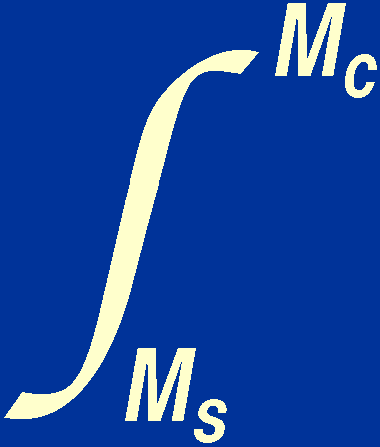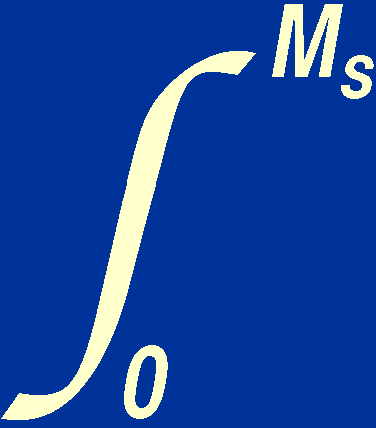Even though we have derived only two of the equations of stellar structure and we have not yet looked at the chemical composition or the physical state of the material of which stars are composed, we can still determine a minimum value for the central pressure of a star. Dividing the equation of hydrostatic support by the equation of mass conservation, we obtain:

(dP / dr) / (dM / dr) = (- GM/ r 2) / 4r 2.

On rearranging, we have:

dP / dM = - GM / 4r 4.

This equation can be written in integral form as:

Pc - Ps = -(GM / 4r 4) dM,

where the subscripts c and s refer to the centre and surface of the star, respectively. By swapping the integration limits and noting that the mass of the star must increase outwards from zero at the centre of the star, i.e. Mc = 0, we can write:

Pc - Ps =(GM / 4r 4) dM.

We can obtain a lower limit to the value of the integral on the right-hand side by noting that, at all points inside a star, r < rs. Hence 1/r > 1/rs and 1/r 4 > 1/rs4. So:(GM / 4r 4) dM   >(GM / 4rs4) dM   =   GMs2 / 8rs4.

Hence

Pc - Ps > GMs2 / 8rs4.

If we approximate the pressure at the surface of the star to be zero, i.e. Ps = 0, we obtain:

Pc > GMs2 / 8rs4.

We know the values of Ms and rs for the Sun and these can be inserted into the inequality to give:

Pc> 4.5 × 1013 Nm-2 = 4.5 × 108 atmospheres.

In light of this high value for the central pressure, it may seem surprising that the solar material is gaseous. As we shall see shortly, it is not an ordinary gas.